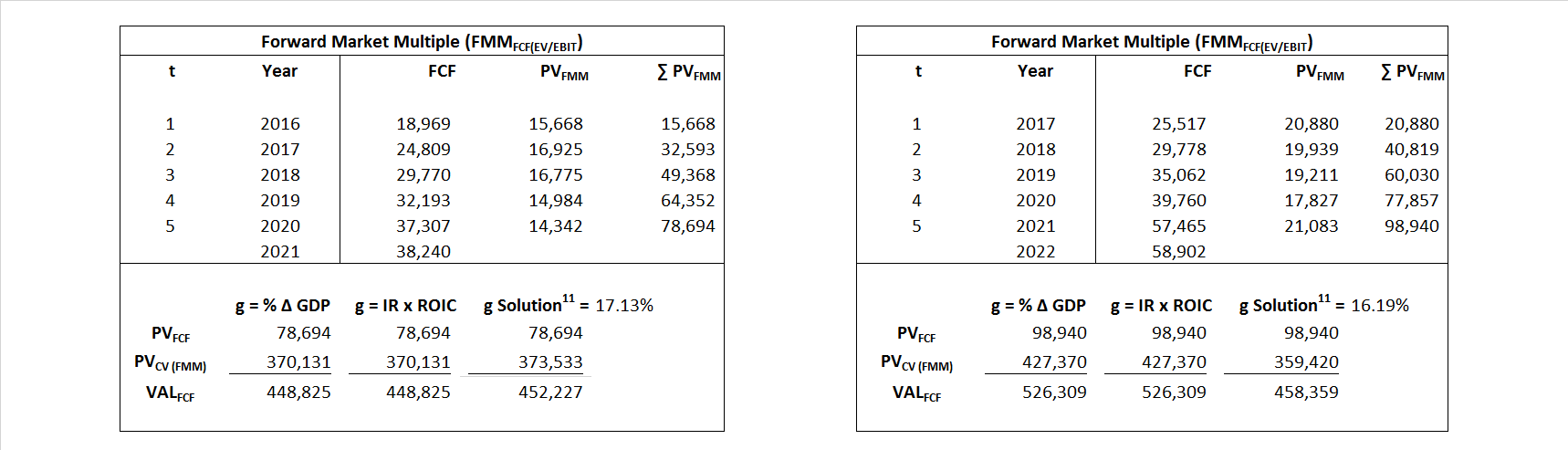## Analyst Listing

The following analysts provide coverage for the subject firm as of May 2016:

 Broker Analyst Analyst Email Bernstein Research Carlos Kirjner carlos.kirjner@bernstein.com Mizuho Securities USA Neil A. Doshi neil.doshi@us.mizuho-sc.com Monness Crespi Hardt Cengiz M. Cakmak jcakmak@mchny.com Nomura Research Anthony DiClemente anthony.diclemente@nomura.com Canaccord Genuity Michael Graham mgraham@canaccordgenuity.com Axiom Capital Victor Anthony vanthony@axiomcapital.com Piper Jaffray Gene Munster gene.a.munster@pjc.com Jefferies Brian Pitz bpitz@jefferies.com Pacific Crest Securities-KBCM Evan Wilson ewilson@key.com Evercore ISI Ken Sena ken.sena@evercoreisi.com Pivotal Research Group Brian Wieser brian@pvtl.com Guggenheim Securities Jake Fuller james.fuller@guggenheimpartners.com FBN Securities Shebly Seyrafi sseyrafi@fbnsecurities.com RBC Capital Markets Mark S. Mahaney mark.mahaney@rbccm.com Cantor Fitzgerald Youssef H. Squali ysquali@cantor.com Atlantic Equities James Cordwell j.cordwell@atlantic-equities.com Susquehanna Financial Group Shyam Patil shyam.patil@sig.com Cowen & Company John Blackledge john.blackledge@cowen.com SunTrust Robinson Humphrey Robert S. Peck robert.peck@suntrust.com JMP Securities Ronald V. Josey rjosey@jmpsecurities.com B Riley & Co Sameet Sinha ssinha@brileyco.com Wells Fargo Securities Peter Stabler peter.stabler@wellsfargo.com Wedbush Securities James Dix james.dix@wedbush.com Hilliard Lyons Stephen Turner sturner@hilliard.com Stifel Nicolaus Scott W. Devitt swdevitt@stifel.com Oppenheimer Jason Helfstein jason.helfstein@opco.com Needham Kerry Rice krice@needhamco.com Daiwa Securities Co. Ltd. Kazuya Nishimura kazuya.nishimura@us.daiwacm.com Raymond James Aaron Kessler aaron.kessler@raymondjames.com William Blair Ralph Schackart rschackart@williamblair.com BMO Capital Markets Daniel Salmon dan.salmon@bmo.com Credit Suisse Stephen Ju stephen.ju@credit-suisse.com Deutsche Bank Research Ross Sandler ross.sandler@db.com BGC Financial, L.P. Colin W. Gillis cgillis@bgcpartners.com

## Primary Input Data## Derived Input Data

### Equational Form

Net Operating Profit Less Adjusted Taxes NOPLAT  11,675   15,065$NOPLAT\, =\, EBIT\, x\, (1 \,-\, Avg \,\,Tax\,\, Rate\,\, on\,\, EBIT)$
Free Cash Flow FCF  16,109  25,824$FCF\,=NOPLAT\,+\,Non-Cash\,Expenses-\Delta NWC\,-\,NCS$
Tax Shield TS  (28)  24$TS\,=\,Interest\,\,Paid\,\,x\,\, Avg \,\,Tax\,\,Rate\,\, on\,\, Pre-Tax\,\, Income$
Invested Capital IC  128,151  150,741$IC\,=\,Fixed\,\,Operating\,\,Assets\,\,+\,\,Net\,\, Working\,\, Capital$
Return on Invested Capital ROIC 9.11% 9.99%$ROIC\,=\,\frac { NOPLAT }{ IC }$
Net Investment NetInv  18,886  28,734$NetInv\,=\,{ {IC}_{1}}-{{IC}_{0}}+Depreciation$
Investment Rate IR 161.77%  190.73%$IR\,=\,\frac {NetInv}{NOPLAT}$
Weighted Average Cost of Capital WACCMarket 21.07% 22.21%$WACC\,=\,\frac { E }{ V } { R }_{ E }\,+\,\frac { P }{ V } { R }_{ P }\,+\,\frac { D }{ V } { R }_{ D }\left( 1- Avg\,\, Tax\,\,Rate\,\,on\,\,Pre-Tax\,\,Income \right)$
WACCBook  10.46% 10.12%
Enterprise value EVMarket  452,227 458,359$EV\,=\,Market\,\,Cap\,\,Equity\,+\,\,Long\,\,Term\,\,Debt\,-\,Cash$
EVBook  451,097  458,261
EV/EBIT Multiple$\frac{EV_{Market}}{EBIT}$  25.18  19.78$EV/EBIT\,=\,\frac { EV}{ EBIT}$
Long-Run Growth g = IR x ROIC
14.74%   19.06% Long-run growth rates of the income variable  are used in the Continuing Value portion of the valuation models.
g = %$\Delta$ GDP    2.50%   2.50%

## Valuation Model Outcomes

The outcomes presented in this study are the result of original input data, derived data, and synthesized inputs and, depending on the equational form of any particular valuation model, may result in irrelevant or implausible results.  For example, in the event WACC < g, the value of this term, often found in the denominator of an equation’s continuation value term, will be expressly negative and may result in a negative overall valuation for the firm.  In the event of a WACC < g relation, the model form as applied to the subject firm offers an irrelevant outcome.

### Equational form

Key Value Driver (NOPLAT) KVD (NOPLAT)${ Value }_{ DCF/KVD }=\sum { \frac { NOPLAT_{ t } }{ { \left( 1+WACC \right) }^{ t } } +\frac { \frac { { NOPLAT }_{ 1 }\left( 1-\frac { g }{ ROIC } \right) }{ WACC-g } }{ { \left( 1+WACC \right) }^{ t } } }$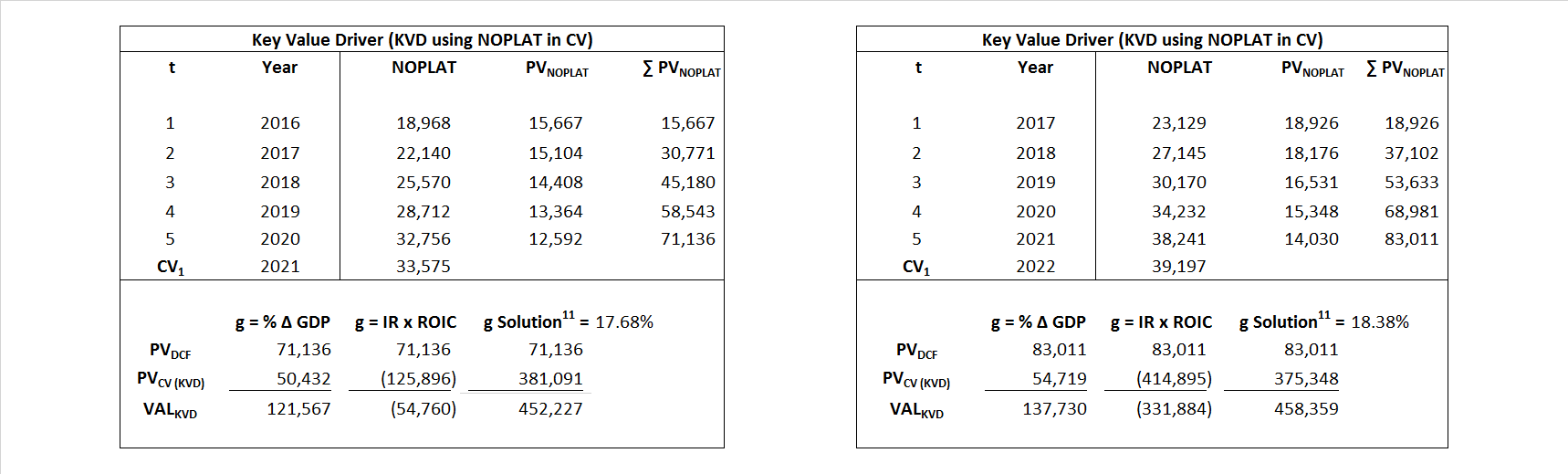Key Value Driver (FCF) KVD (FCF)${ Value }_{ DCF/KVD }=\sum { \frac { FCF_{ t } }{ { \left( 1+WACC \right) }^{ t } } +\frac { \frac { { NOPLAT }_{ 1 }\left( 1-\frac { g }{ ROIC } \right) }{ WACC-g } }{ { \left( 1+WACC \right) }^{ t } } }$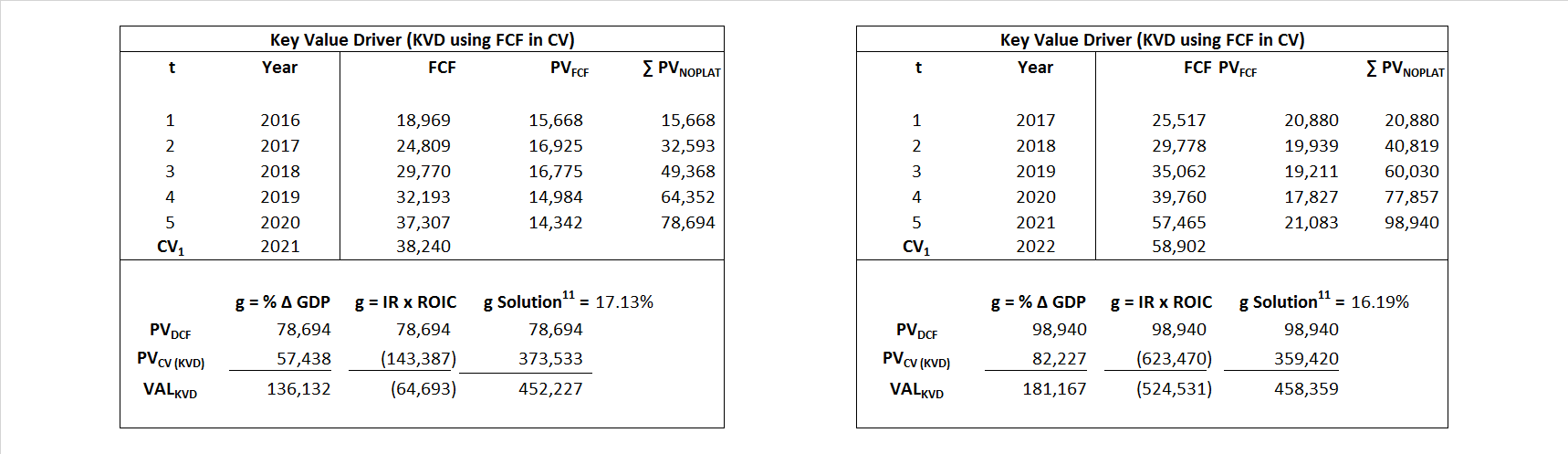Free Cash Flow FCF${ Value }_{ DCF/FCF }=\sum { \frac { FCF_{ t } }{ { \left( 1+WACC \right) }^{ t } } +\frac { \frac { { FCF }_{ 1 }}{ WACC-g } }{ { \left( 1+WACC \right) }^{ t } } }$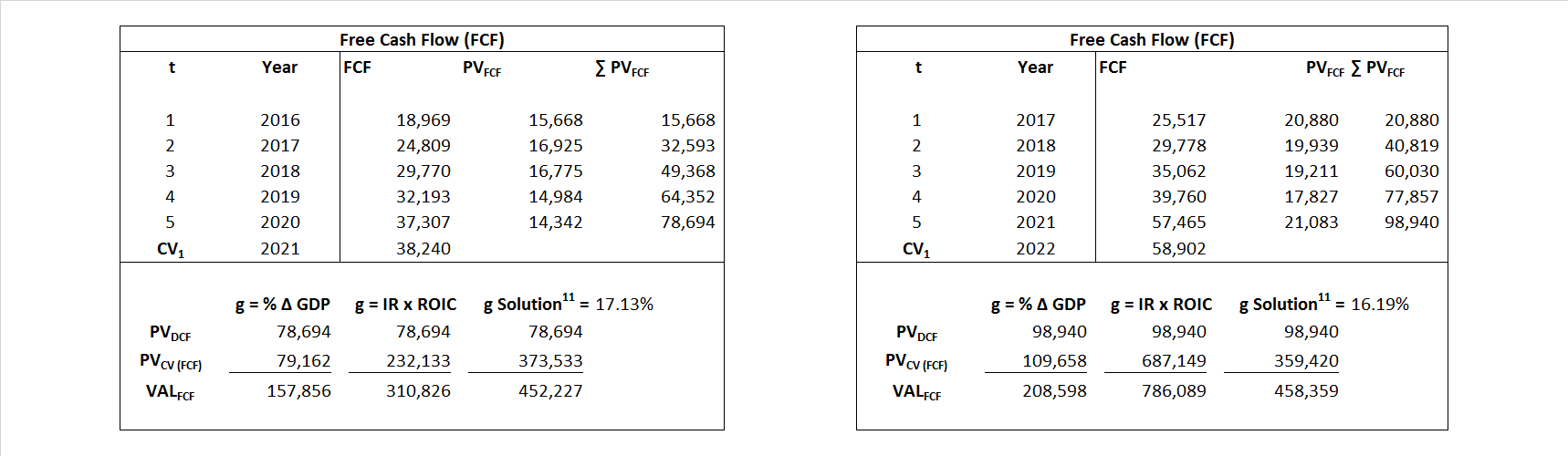Economic Profit ECON π${ Value }_{ { ECON\pi } }= I{ C }_{ 0 }+\sum { \frac { { IC }_{ t-1 }(ROI{ C }_{t}-WAC{C}_{t}) }{ { \left( 1+WACC \right) }^{ t } }+ \frac {\frac { I{C}_{0}\ x\ (ROI{C}_{1}\ -\ WAC{C}_{1}) }{ WACC-g } }{ { \left( 1+WACC \right) }^{ t } } }$Adjusted Present Value APV${ Value }_{ APV }=\sum { \frac { FCF_{ t } }{ { \left( 1+{ k }_{ u } \right) }^{ t } } +\frac { \frac { { FCF }_{ 1 }}{ { k }_{ u }-g } }{ { \left( 1+{ k }_{ u } \right) }^{ t } } } +\sum { \frac { { TS }_{ t } }{ { \left( 1+{ k }_{ tax } \right) }^{ t } } +\frac { \frac { { TS }_{ 1 }}{ { k }_{ tax }-g } }{ { \left( 1+{ k }_{ tax } \right) }^{ t } } }$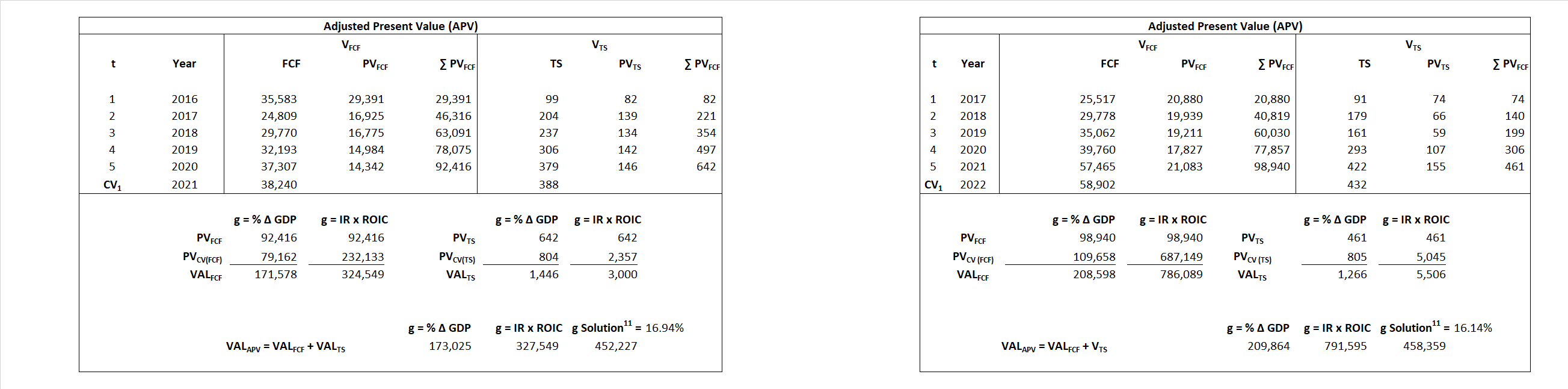Forward Market Multiple FMM${ Value }_{ DCF/FMM}=\sum { \frac { FCF_{ t } }{ { \left( 1+WACC \right) }^{ t } } +\frac { { EBIT }_{ 1 }\,{x}\,{FMM}}{ { \left( 1+WACC \right) }^{ t } } }{\,\,\,; \,\,FMM\,=\,\frac{{EV}_{t=0}}{{EBIT}_{t=0}}}$